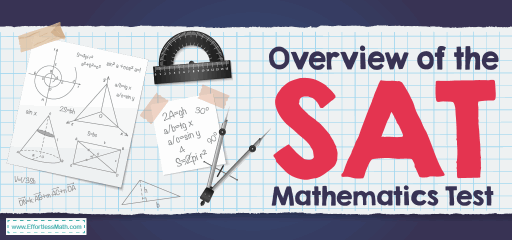# Overview of the SAT Mathematics TestThe SAT is an entrance test used by most universities and colleges to decide on admission. The purpose of the SAT is to measure the readiness of a high school student to study at university and also to provide a common data point to colleges that can be used to compare all applicants.

The SAT is a pencil and paper, and a multiple-choice test created and managed by a college board. The SAT consists of three main parts: Math, Evidence-Based Reading, and Writing. The total time for SAT is 180 minutes, not including breaks.

## SAT Math format

Mathematics is the third and fourth sections of the SAT. You will first receive 15 multiple choices and 5 grid-ins questions that you have 25-minute to answer. During which you cannot use the calculator. After a short rest, you will move on to the 55-minute section that has 30 multiple choices and 8 grid-ins questions. In this long section, you are allowed to use your calculator.

Both sections begin with multiple-choice questions, each with four answer options. You will be asked for some student-produced responses, commonly known as grid-ins.

The College Board divides question types into three main categories: Passport to Advanced Math, Heart of Algebra, and Problem Solving and Data Analysis. These three areas describe 90% of the SAT math questions. The remaining 10% are called Additional Topics.

### Heart of Algebra:

SAT math questions in the Heart of Algebra category includes:

• Solving linear equations and linear inequalities
• Interpreting linear functions
• Linear inequality and equation word problems
• Graphing linear equations
• Linear function word problems
• Systems of linear inequalities word problems
• Solving systems of linear equations

The questions of this section include:

• Interpreting nonlinear expressions
• Quadratic and exponential word problems
• Operations with rational expressions and polynomials
• Polynomial factors and graphs
• Nonlinear equation graphs
• Structure in expressions
• Isolating quantities
• Functions

### Problem Solving and Data Analysis:

The questions of this section consist of:

• Ratios, rates, and proportions
• Percent
• Units
• Table data
• Scatterplots
• Key features of graphs
• Linear and exponential growth
• Data inferences
• Center, spread, and shape of distributions
• Data collection and conclusions

These topics include:

• Volume word problems
• Right triangle word problems
• Congruence and similarity
• Right triangle geometry
• Angles, arc lengths, and trig functions
• Circle theorems
• Circle equations
• Complex numbers

## Is the SAT mathematics test hard?

The SAT will not be too hard on the math front if you understand the concepts, practice well, and solve the sample questions.

## Do you get a formula sheet on the SAT math test?

You will be given 12 necessary formulas and three rules of geometry formulas. However, the test does not provide a list of all the basic formulas needed to know the SAT math. It means that you need to recall many mathematical formulas in the SAT.

## Can you use a calculator on the SAT math test?

The SAT calculator policy allows test takers to use the calculator in certain parts of the test but does not provide them.

## How is theSAT math test score?

You can earn a scaled score of between 200 and 800 points in the math section.

## College Entrance Tests

Looking for the best resource to help you succeed on the SAT Math test?

## The Best Books to Ace the SAT Math Test

### What people say about "Overview of the SAT Mathematics Test - Effortless Math: We Help Students Learn to LOVE Mathematics"?

No one replied yet.

X
49% OFF

Limited time only!

Save Over 49%

SAVE \$34

It was \$69.99 now it is \$35.99